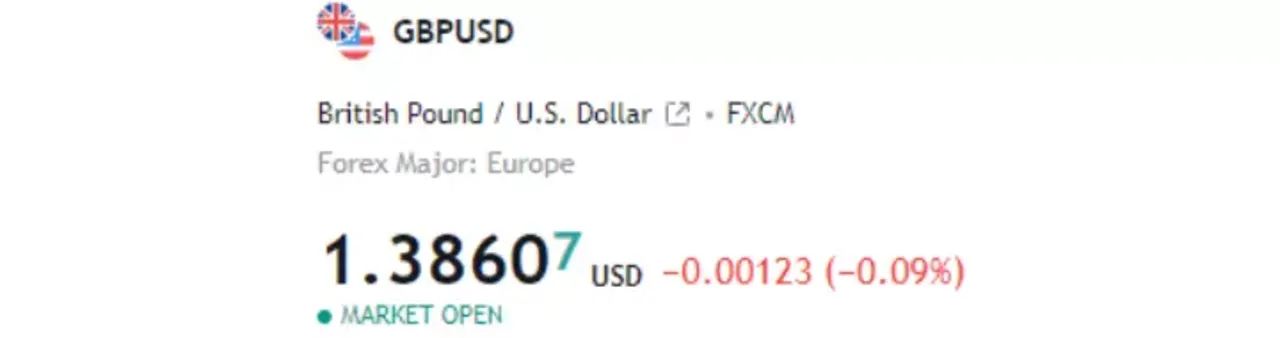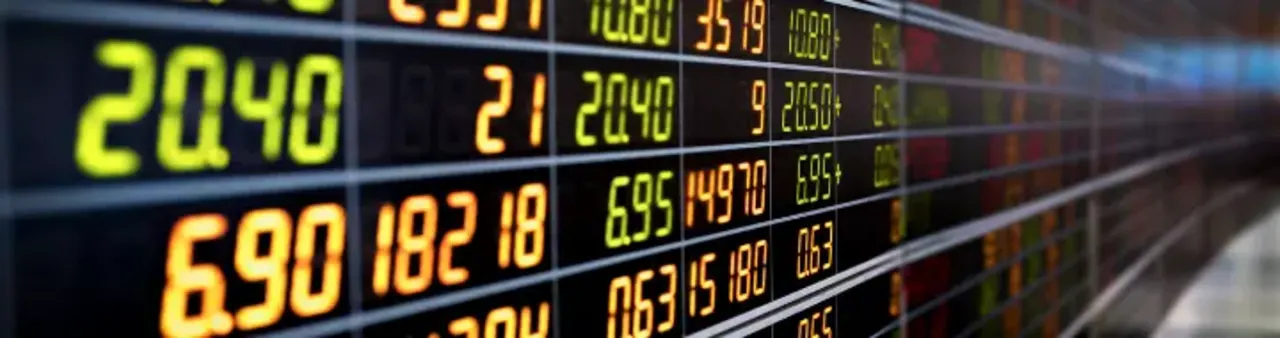# What is a pip?

You might have heard forex traders saying that they profited 100 pips on a single trade but do you actually know what a pip is?

Even if you know what a pip is exactly, do you know why we have pips in the first place? Don’t worry, this blog post has all answers.

In this blog post, I will tell you everything you need to know about pips, right from what it is to why we have pips in forex.

In the end, I will show you how you can calculate the value of each pip which will make it easy for you when you actually go on to take trades, so stick till the end.

### Contents

What is a pip?

Why pip?

What is a pipette?

How much is a pip worth?

## What is a pip?Pip is short for Point in Percentage. A pip represents a minimum amount of change that can take place in the price of a currency pair. It is the unit measure used by forex traders to determine price change in currency pairs.

In forex, a pip represents the 4th decimal point of a currency pair’s price quote. Hence when the price of a currency pair increases or decreases by 1 pip, it means that the 4th decimal point of the price quote increased or decreased by 1 basis point or pip.

Let us consider the currency pair of USDEUR, quote price of 0.8500. If the price of the currency pair moves to 0.8501 or 0.8499, the price is said to increase by 1 pip or decrease by 1 pip respectively.

Similarly, a 10 pip movement will be when the 3rd decimal place of the price quote of the currency pair increases or decreases.

Going by the above-mentioned example, if the price of the currency pair moves to 0.8510 or 0.8490, then the price of the currency pair is said to move by 10 pips. A 100 pip movement will be when the 2nd decimal place of the quote changes and a 1000 pip movement will indicate that the 1st decimal place of the price quote of the currency pair either increased or decreased.

In all currency pairs, 1 pip movement indicates a movement of the 4th decimal place of the price quote. Only currency pairs including the JPY, Japanese Yen, have pip movements as price change in the 2nd decimal place of the price quote.

## Why pip?In forex, the currencies that are listed cannot be traded standalone. Currencies are traded in pairs. These pairs are made up of two currencies.

The first being the base currency and the other being the quote currency. If a trader wants to trade in a currency, say USD, he will have to either buy or sell a currency pair with USD as the base currency, say USDEUR.

The price quote of the currency pair that we see on trading terminals is not the price of the base currency, but it indicates how much the base currency is worth in terms of the quote currency

Since there are so many currencies on the forex market and hence so many currency pairs with different currencies as quote currencies, there is no uniform unit of change for the price movements. Hence traders labeled each price movement as pips.

## What is a pipette?A pip indicates a price change of 1/100th of 1% i.e. the 4th decimal place. If this was not already a confusing unitary measure of price change, the pip has been further broken down into pipettes.

A pipette is 1/10th of a pip. This means that if the price of a currency pair is said to have changed by 1 pipette, the 5th decimal place of the price quote of the currency pair has either increased or decreased.

For instance, the quote price of the USDEUR currency pair is 0.86500, the price is said to move by 1 pipette if the price increases to 0.86501 or decreases to 0.86499.

Pipettes are also known as fractional pips or points.

## How much is a pip worth?We know that forex trading is done in currency pairs and not in a single currency. Hence for every currency pair, a pip will have different values.

It is important to calculate how much each pip is worth because it is the risk that traders will take in their positions.

If the trader knows the value of each pip, he can size his position according to his risk limits. If the trader is not aware of how much each pip is worth then it could lead to having heavier positions, risking a lot, or having a small size, hence risking very little on the trade.

The basic calculation of determining the value of each pip is to divide 1 pip by the price quote of the currency pair. This will give the trader the value of each pip in terms of the base currency.

For instance, USDEUR pair, quote price - 0.8680. This is read as 1 USD = 0.8680 EUR. To find the value of each pip we have to divide 1 pip i.e. 0.0001 by the quote price i.e. 0.8680, hence 0.0001/0.8680 = 0.00012.

This means that if a trader trades 1 unit of the USDEUR currency pair each pip movement will either cost him \$ 0.00012 or will profit him that much. It should be noted that this is applicable if the trader trades just 1 unit of the currency pair. But this is not the case in actual forex trading.

Traders buy or sell currency in lots. Traders have the option of choosing between different lot types according to its size.

There is a standard lot that consists of 100,000 units of the currency, and then there is a mini lot that consists of 10,000 units of the currency.

There are also micro-lots, consisting of 1,000 units of the currency, and nano lot that is of 100 units of the currency.

Once the trader calculates the pip value according to the calculation illustrated above, he is then supposed to multiply that value with the position size he is carrying.

This will then give him exact figures of how much each pip movement will affect his profit and loss for the entire position.